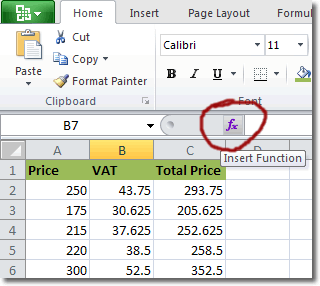# Planet For Application Life Development Presents MY IT WorldExplore and uptodate your technology skills...

Excel - Function

A function in Excel calculates a result based on one or more input values. For example, the SUM function returns the sum of all the cells you specify as arguments. If you were to type into a cell the following:
``` =SUM(B2:B6) ```
then SUM is the function, B2:B6 are the arguments and the whole thing is a formula.

SUM is a straightforward function, but what if you want to use a function whose name you don’t know. Or perhaps you know the function’s name, but don’t know what arguments it needs. This is where the Insert Function command can help. The Insert Function button is located in the formula bar.When you click on this button, the Insert Function dialogue box is presented, which you can use to search for information about the function you require.If you type into the top input box a description of what your function needs to do and then click Go, Excel will do its best to retrieve a match. There is also a dropdown list that you can use to select a category for the functions you used most recently.

If you select a particular function in the list, the following details are displayed about it in the space below:

• the function’s name
• the arguments that the function expects
• a description of what the function does

For example, in the selected function below, the functions name is PMT, it needs 5 input values and it calculates loan payments.If you require more help on how to use the function, you can click on the Help on this function link at the bottom of the window. Doing so will open Excel’s help panel and display details about the selected function.

## Commonly Used Excel Functions

Excel 2010 comes with many built in functions that cover a wide range of topics. Some of the more commonly used ones are given below.

• ABS: Returns the absolute value of a number
• AVERAGE: Adds its arguments
• CONCATENATE: Joins several text items into one text item
• COUNT Counts how many numbers are in the list of arguments
• Date: Returns the serial number of a particular date
• Day: Converts a serial number to a day of the month
• Hour: Converts a serial number to an hour
• Rounds a number down to the nearest integer
• ISBLANK: Returns TRUE if the value is blank
• MAX: Returns the maximum value in a list of arguments
• MIN: Returns the minimum value in a list of arguments
• Minute: Converts a serial number to a minute
• Month: Converts a serial number to a month
• Now: Returns the serial number of the current date and time
• PRODUCT: Multiplies its arguments
• Second: Converts a serial number to a second
• SUM: Adds its arguments
• Today: Returns the serial number of today’s date